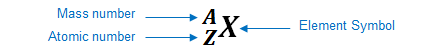# Problem: An element forms an ionic compound with chlorine, leading to a compound having the formula XCl2. The ion of element X has mass number 89 and 36 electrons. Identify the element X, tell how many neutrons it has, and name the compound.

###### FREE Expert Solution

We’re being asked to determine the atomic number of an atom that has a mass number of 27 and 14 neutrons

Recall: an element can be represented as:You can determine the identity of the element using its atomic number:

Atomic number (Z):

84% (499 ratings)###### Problem Details

An element forms an ionic compound with chlorine, leading to a compound having the formula XCl2. The ion of element X has mass number 89 and 36 electrons. Identify the element X, tell how many neutrons it has, and name the compound.